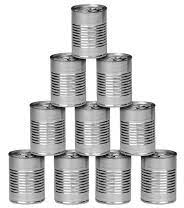# Confirmed 80770

The merchant ordered 200 glasses, and the order was confirmed with the fact that he will receive 41 in rabic, packed in 4 and 6 pieces. How many boxes of 4 and how many boxes of 6?

a =  23
b =  18

### Step-by-step explanation:

41=a+b
200 = 4a+6b

41=a+b
200 = 4·a+6·b

a+b = 41
4a+6b = 200

Pivot: Row 1 ↔ Row 2
4a+6b = 200
a+b = 41

Row 2 - 1/4 · Row 1 → Row 2
4a+6b = 200
-0.5b = -9

b = -9/-0.5 = 18
a = 200-6b/4 = 200-6 · 18/4 = 23

a = 23
b = 18

Our linear equations calculator calculates it.Did you find an error or inaccuracy? Feel free to write us. Thank you!

Tips for related online calculators
Do you have a system of equations and looking for calculator system of linear equations?